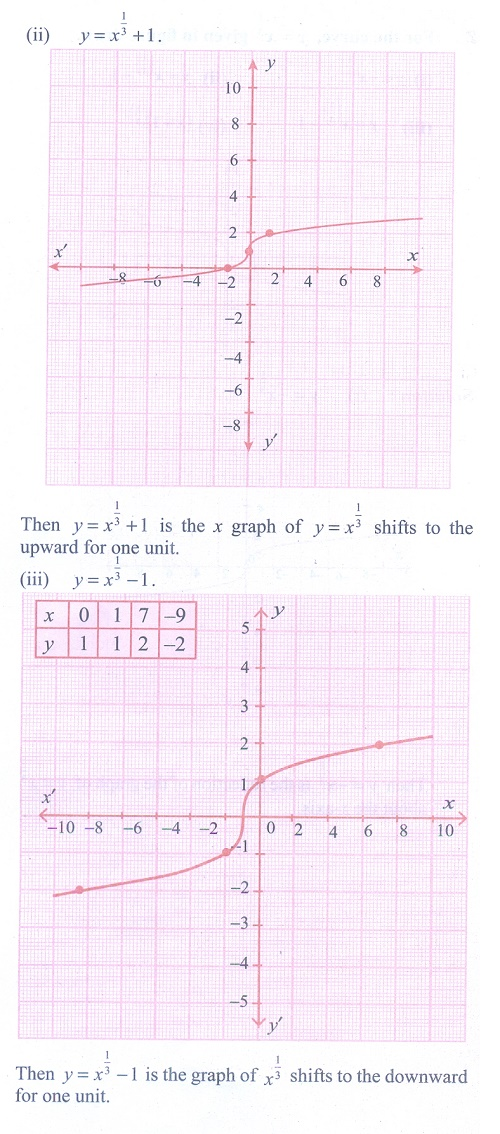Home | | Maths 11th std | Exercise 1.4: Graphing Functions using Transformations

# Exercise 1.4: Graphing Functions using Transformations

Maths Book back answers and solution for Exercise questions - Mathematics : Sets, Relations and Functions: Graphing Functions using TransformationsTags : Problem Questions with Answer, Solution | Mathematics , 11th Mathematics : UNIT 1 : Sets, Relations and Functions
Study Material, Lecturing Notes, Assignment, Reference, Wiki description explanation, brief detail
11th Mathematics : UNIT 1 : Sets, Relations and Functions : Exercise 1.4: Graphing Functions using Transformations | Problem Questions with Answer, Solution | Mathematics